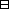## Group Theory and Linear Algebra

Last updated: 24 September 2014

## Lecture 30: Matching the affine orthogonal group with isometries

Define $Φ: AOn(ℝ) ⟶ Isom(𝔼n) y ⟼ fy where$ $fy( 0 x 0 1 ) = 0 0 0 0 0 g 0 μ 0 0 0 0 0 0 0 1 0 x 0 1 if y= 0 0 0 0 0 g 0 μ 0 0 0 0 0 0 0 1 .$ Then $\mathrm{\Phi }$ is a group isomorphism.Proof.

To show:
 (a) $\mathrm{\Phi }$ is a function ($\mathrm{\Phi }$ is well defined). (b) $\mathrm{\Phi }$ is a group homomorphism. (c) $\mathrm{\Phi }$ is a bijection.
(b) If $y,z\in A{O}_{n}\left(ℝ\right)$ and $\left(\begin{array}{c}\phantom{0}\\ x\\ \phantom{0}\\ 1\end{array}\right)\in {𝔼}^{n}$ then $fyfz 0 x 0 1 = yz 0 x 0 1 = fyz 0 x 0 1 .$ So $\mathrm{\Phi }$ is a homomorphism.
(a) To show: If $y\in A{O}_{n}\left(ℝ\right)$ then ${f}_{y}$ is an isometry.
Assume Then ${f}_{y}={t}_{\mu }g$ where $tμ: 𝔼n ⟶ 𝔼n 0 x 0 1 ⟼ 0 μ+x 0 1 is a translation,$ and If $x,z\in {𝔼}^{n}$ then $d(tμx,tμz) = d(μ+x,μ+z) = ⟨(μ+x)-(μ+z), (μ+x)-(μ+z)⟩ = ⟨x-z,x-z⟩ = d(x,z)$ and so that $d(gx,gz) = ⟨gx-gz,gx-gz⟩ = ⟨g(x-z),g(x-z)⟩ = ⟨x-z,x-z⟩ = d(x,z).$ Thus $g,{t}_{\mu }$ and ${f}_{y}={t}_{\mu }g$ are all isometries.
(c) To show: There is an inverse function to $\mathrm{\Phi }.$
Define $Ψ: Isom(𝔼n) ⟶ AOn(ℝ) f ⟼ 0 0 0 0 0 g 0 μ 0 0 0 0 0 0 0 1$ where $μ=f(0)$ and where
To show:
 (ca) $\mathrm{\Psi }$ is well defined. (cb) $\mathrm{\Psi }\circ \mathrm{\Phi }={\mathrm{id}}_{A{O}_{n}}$ and $\mathrm{\Phi }\circ \mathrm{\Psi }={\mathrm{id}}_{\mathrm{Isom}}.$
 (cb) Let $\left(\begin{array}{cccc}\phantom{0}& \phantom{0}& \phantom{0}& \phantom{0}\\ \phantom{0}& g& \phantom{0}& \mu \\ \phantom{0}& \phantom{0}& \phantom{0}& \phantom{0}\\ \phantom{0}& 0& \phantom{0}& 1\end{array}\right)\in A{O}_{n}.$ Then $(Ψ∘Φ) 0 0 0 0 0 g 0 μ 0 0 0 0 0 0 0 1 = Ψ(fy) = 0 0 0 0 0 g' 0 μ' 0 0 0 0 0 0 0 1$ where $g'= | | | g1' g2' ⋯ gn' | | | with gj' = fy(ej) = 0 0 0 0 0 g 0 μ 0 0 0 0 0 0 0 1 0 ⋮ 1 0 ⋮ 0 1 = | gj | 1 = | gj |$ and $μ'=fy(0) with fy(0) = 0 0 0 0 0 g 0 μ 0 0 0 0 0 0 0 1 0 ⋮ 0 1 = 0 μ 0 1 =μ.$ So $(Ψ∘Φ) 0 0 0 0 0 g 0 μ 0 0 0 0 0 0 0 1 = 0 0 0 0 0 g 0 μ 0 0 0 0 0 0 0 1 .$ (ca) Let $f\in \mathrm{Isom}.$ Then $(Φ∘Ψ)(f) = Φ 0 0 0 0 0 g 0 μ 0 0 0 0 0 0 0 1 = fy where y= 0 0 0 0 0 g 0 μ 0 0 0 0 0 0 0 1$ and $g= | | g1 ⋯ gn | | with f(ej) = | gj | = g 0 ⋮ 0 1 0 ⋮ 0 =gej,$ and $\mu =f\left(0\right).$ To show: $f\left(\begin{array}{c}\phantom{0}\\ x\\ \phantom{0}\\ 1\end{array}\right)={f}_{y}\left(\begin{array}{c}\phantom{0}\\ x\\ \phantom{0}\\ 1\end{array}\right).$ To show: ${t}_{-\mu }f={t}_{-\mu }{f}_{y}.$ Let $g={t}_{-\mu }{f}_{y}.$ Let $h={t}_{-\mu }f.$ To show: If $x\in {𝔼}^{n}$ then $hx=gx.$ We know $h\in \mathrm{Isom}\left({𝔼}^{n}\right)$ and $h\left(0\right)=0.$ If $x,z\in {𝔼}^{n}$ then, since $h\left(0\right)=0,$ $⟨hx,hz⟩ = 1 2 ( ⟨hx,hx⟩+⟨hz,hz⟩-⟨hx-hz,hx-hz⟩ ) = 1 2 ( d(hx,0)2 + d(hz,0)2 - d(hx,hz)2 ) = 1 2 ( d(hx,h0)2 + d(hz,h0)2 - d(hx,hz)2 ) = 1 2 ( d(x,0)2 + d(z,0)2 - d(x,z)2 ) = 1 2 ( ⟨x,x⟩ + ⟨z,z⟩ - ⟨x-z,x-z⟩ ) = ⟨x,z⟩.$ Assume $x=\left(\begin{array}{c}{x}_{1}\\ ⋮\\ {x}_{n}\\ 1\end{array}\right)\in {𝔼}^{n}.$ Since $h{e}_{i}=g{e}_{i},$ So $hx=gx.$

$\square$

## Notes and References

These are a typed copy of Lecture 30 from a series of handwritten lecture notes for the class Group Theory and Linear Algebra given on October 14, 2011.